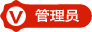﻿ 用户定义的类型（VFB教程2-5）-编程-勇芳软件开发小组用户定义的类型（VFB教程2-5）2018-2-16 4788

'Define a UDT called myType, with an Integer member named someVar
Type myType
As Integer someVar
End Type

'Create a variable of that type
Dim myUDT As myType

'Set the member someVar to 23, then display its contents on the screen
myUDT.someVar = 23
Print myUDT.someVar

UDT指针

Type rect
x As Integer
y As Integer
End Type

Dim r As rect
Dim rp As rect Pointer = @r

rp->x = 4
rp->y = 2

Print "x = " & rp->x & ", y = " & rp->y
Sleep

Type float As Single

Dim foo As float = 1.23
Dim bar As float = -4.56

Type func_t As Function (ByRef As String) As Integer

Dim func As func_t = @f

Function f (ByRef arg As String) As Integer
Function = CInt(arg)
End Function

Type float As Single

Declare Sub f Overload (a As Single)

'' If uncommented, this will generate a duplicated definition error
'' Declare Sub f (a As float)

Dim pf As Function() As Integer Ptr

Type pf_t As Function() As Integer
Dim ppf As pf_t Ptr

Type foo As bar

Type sometype
f   As foo Ptr
End Type

Type bar
st  As sometype
a   As Integer
End Type

Type list As list_

Type listnode
parent As list Ptr
text As String
End Type

Type list_
first As listnode Ptr
count As Integer
End Type

Type sometype As sometype_

'' Not allowed since size of sometype is unknown
'' TYPE incomplete
''   a AS sometype
'' END TYPE

'' Allowed since size of a pointer is known
Type complete
a As sometype Ptr
End Type
Dim x As complete

'' Not allowed since size of sometype is still unknown
'' DIM size_sometype AS INTEGER = SIZEOF( sometype )

'' Complete the type
Type sometype_
value As Integer
End Type

'' Allowed since the types are now completed
Dim size_sometype As Integer = SizeOf( sometype )

Type completed
a As sometype
End Type

Dim size_completed As Integer = SizeOf( completed )